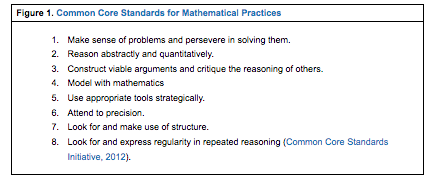Oak Grove Curriculum Scope & Sequence

Math (Grade 1)

Updated 2.10.17

____ Major Cluster (70%)        ____ Supporting Cluster (20%)        ____ Additional Clusters (10%)Unit Length(32 Wks) Unit / SkillsWe are learning to…Skill (standard) Instructional Strategies Resources AssessmentsFormative/Summative (F/S) Ongoing through- out year Problem SolvingWe are learning to…Solve for an unknown in any position (OA.1, 4) Use multiple math models to represent and solve problems (OA.1, 2)Write and compare equations and expressions (OA.3, NBT.3) Direct InstructionWork PlacesMath Talk number racks5,10, 20 framesUnifix cubesBar models Homework (F)Quizzes (Checkpoints)  (F)Teacher Made Assessment (S) 5-6 weeks Unit 1We are learning to...Explore math manipulatives (OA.1,2)Develop number sense (OA.5, NBT.1, NBT.2a,NBT.2b,NBT.2c,NBT.5)Collect and organize data to recognize patterns (MD.4)Develop number combinations to 10 and reinforce number combinations of 5 (OA.6)Recognize number relationships (fact families, part/part/whole) (OA.1, OA.2) (OA.3, OA.4)Use mathematical models  (OA.1, OA.2)Measure with nonstandard units (MD.1, MD.2)Subitize quantities and add 1 and subtract 1 Explain our math thinking (MP) Routine buildingExploring and communicating about numbersDevelop visual imageryDifferentiate up to 20 or down to 5 as needed and appropriateSubitize flashingWork PlacesMath Talk math manipulatives of: geoboards, pattern blocks, links, polydrons, dominoesnumber racks5 and 10 framespopsicle sticks, unifix cubes, heel to toe steps Homework (F)Quizzes (Checkpoints)  (F)Teacher Made Assessment (S) 5-6 weeks Unit 2We are learning to...Write equations (OA.1, OA.2, OA.8)Write inequalities statements (OA.7)Add and subtract single digit numbers (OA.1, OA.6)Use a variety of strategies to add and subtract including: (OA.5, OA.6) Counting on and counting back  Combining small groups of numbers within larger numbers (subitizing) Building from known factsUsing double factsCounting by 5 and 10’sUsing the commutative propertyUse and generate fact familiesImprove our efficiency by counting groups using 5’s(_____) and 10’s (NBT2.a)View the equal sign as a way to indicate that two expressions are of equal value (OA.7, OA.8)Explain our math thinking(MP) Work PlacesMath Talk dominoesdot cardsnumber racksdouble flap cards (for addition and subtraction relationship)doubles flap cardsnumber racknickels, dimes, pennies Homework (F)Quizzes (Checkpoints)  (F)Teacher Made Assessment (S) 5-6 weeks Unit 3We are learning to...Develop fluency of key number facts and fact strategies for single-digit addition and subtraction (doubles, near doubles, and sums of 10) (OA.6)Subtract to compare (the difference model of subtraction) (OA.5, OA.6)Use the fact family relationships to solve addition and subtraction problems (OA.6)Use symbols to compare (NBT.3)Use the number rack to:recognize number combinations to 10subitizefind the sum of two numbers compare to find the difference (OA.5, OA.6)Recognize and build numbers from 11 to 20 (using 10 and some more) (NBT.2a, NBT.2b)Build place value understanding by using the make 10 strategy to solve combinations to 20 (0A.6)Write and compare equations and expressions (NBT.3)Explain our math thinking (MP) Work PlacesMath Talk number racksunifix cubes Homework (F)Quizzes (Checkpoints)  (F)Teacher Made Assessment (S) 5-6 weeks Unit 6We are learning to...Explain our math thinking (MP)Add and subtract to to 20 (OA.1, OA.2)Continue to develop fluency with addition and subtraction facts to 10 (OA.1, OA.6)Continue to develop strategies for working with facts to 20 (OA.6, OA.7, OA.8)Write and solve equations that involve unknowns in all positions (OA.3, OA.4, OA.8)Determine whether addition and subtraction equations are true or false(OA.7) Interactive problem solvingMath TalkWork Places Number RackDouble Flap Dot Cards Homework (F)Quizzes (Checkpoints)  (F)Teacher Made Assessment (S) 5-6 weeks Unit 4We are learning to…Use an open number line as a model and a tool (MP)Locate and identify numbers on an open number line (NBT.1)Model addition and subtraction on an open number line (OA.6)Determine missing numbers on an open number line within the range of 0-120 Make interval jumps of 1’s, 5’s and 10’s on an open number on and off the decade        Explain our math thinking (MP) Work PlacesMath Talk life-sized open number line and number cards Homework (F)Quizzes (Checkpoints)  (F)Teacher Made Assessment (S) 4-5 weeks Unit 5We are learning to…Identify, describe, construct, draw, compare, compose and sort 2-D and 3-D shapes (G.1, G.2)Identify 2-D shapes (triangle, trapezoid, squares, rhombuses rectangles and hexagons) (G.1)Compare and distinguish defining attributes (sides, corners/vertices, right angles, straight lines, closed shape, parallel lines) from non-defining attributes (shape, size, color) (G.1)Identify 3-D shapes (cubes, rectangular prism, cones, cylinders, sphere, triangular prism, pyramids) (G.2)Compare and distinguish and sort defining attributes (faces, edges, vertices, flat and curved surfaces, roll, slide) from non-defining attributes (shape, size, color) (G.1)Use nets to compose and decompose cubes and square pyramids (G.2)Identify, name, describe squares (G.1)Build  3-dimensional shapes with specific defining attributes. (G.2)Explore fractions including halves and fourths/quarters (use half of, fourth of and quarter of) (G.3)Explain our math thinking (MP) Work PlacesMath Talk Homework (F)Quizzes (Checkpoints)  (F)Teacher Made Assessment (S)